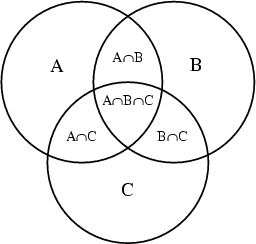# Set theory - Equality

The equality relationship indicates that two sets are equal if they contain exactly the same elements. (There is no order among elements of a set.)

## Notation

Two sets are equal if they contain exactly the same elements. (There is no order among elements of a set.) $$\Large A = B$$

## Proof

A convenient way to prove that A and B are equal is to prove that each is a subset of the other. The proof often consists of two parts:

1. a proof that(ie A subset B) and
2. a proof that(ie B subset A)

Discover MoreSet Theory - Operator (Operations)

operator in Set Theory that applies to set structure (set, bag) A data operator produce another set. All relational algebra operator are set-operator. A relationship operator produce...Set Theory - Relationship

The relationships between set structure is: given by a mathematical function and is described by the set operators. You can visualize them with venn diagram.